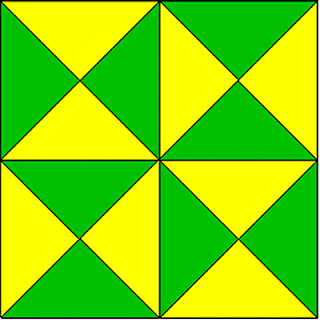# Can You Count

40.0K Views

Count the Triangle in the figure given below:44

Each of the 4 small squares contain 8 triangles each => 4 x 8 = 32
There are Four triangles with the sides of the larger square as base and vertex at the centre. => 4
Also, there are Four more triangles, each formed with two adjacent sides of the larger square and the diagonal of the square forming the third side. => 4
Further,  Four more triangles are there with vertices at the centre of each side of the large square.  => 4

Total: 32 + 4 + 4 + 4 = 44